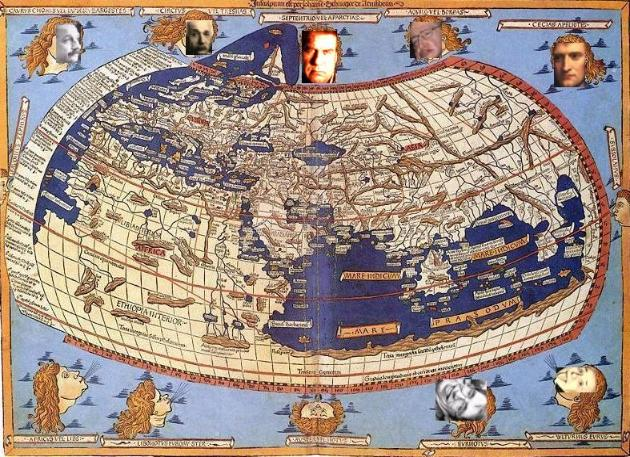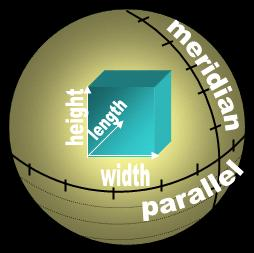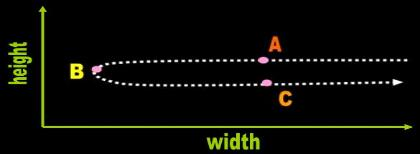The 'number of numbers' definition of dimension cannot be used consistently
 Adapted for the Internet from:Why God Doesn't Exist

1.0   The dimensions of Math are dynamic and curved

Ferris tells us that the Earth is 'unbounded' in two dimensions because we can run around it without
encountering a wall.

" the earth is finite but unbounded in two dimensions (we can wander wherever we
like, and will not fall off the edge of the earth)" (p. 201)  

Ridley explains that a point goes from A to C via location B along something he calls a ‘one-dimensional
space’ (Fig. 1)!  He clarifies that:

"We call the single tramline a one-dimensional space, and the double set of tram-lines a two-
dimensional space..." (p. 43) 

He adds that dimensions represent the degrees of freedom of movement an object has, a notion that is
corroborated in other books and websites.

This is actually quite revealing because, whereas Hawking presented a static scenario to exemplify
dimensions, Ferris and Ridley 'explain' dimensions dynamically. If Hawking confuses dimensions with
coordinates, Ferris and Ridley confuse them for vectors. If we add that Hawking exemplifies his
dimensions with artificial quantities and units – the bread and butter of Mathematics – it is obvious that
relativists are not talking about vectors, coordinates or dimensions at all, but about number lines (Fig. 2).
The mathematicians are not talking about qualitative and physical relations, but about artificial
magnitudes and quantities. 'Mathematical objects' such as meter, kilogram, second, numbers, energy,
force, and field or similar quantifiable parameters and concepts stand in stark contrast to natural,
qualitative, physical attributes such as direction, form, location, angle, parallel, and perpendicular. The
latter cannot be depicted with numbers or variables a priori. Something has shape, is perpendicular, or
forms an angle before we invent numbers and standards. Therefore, relativists cannot hope to explain
the nature of our Universe with their definitions and usages.
 Fig. 1  One-dimensional space?
 Fig. 2   The 3-D cube of Physics within the 2-D sphere of MathSo what we do in these cases, Bill, is bend the dimension!
 The mathematicians have developed a ridiculous and blatantly erroneous notion of dimension. First, the word dimension of Mathematics has nothing to do with architecture (i.e., length, width, and height) It has to do solely with motion. Mathematics is a discipline unconcerned with structure. It exclusively studies dynamic situations. Then, the mathematicians deflect the itinerary of a traveling object and continue labeling it one-dimensional for reasons they only know. The trajectory has encroached on a second 'physical' dimension, but in Math, for unknown reasons, they call it the 2-D. The root of the problem is that the morons of Mathematics confuse straight with forward! In the idiotic religion of Mathematical Physics, anything that travels forward is traveling straight,and as long as you continue traveling forward, you are traveling 'one-dimensionally'!(So Bill? Why is it that you say that the mathematicians of the world are a bunch of stupid idiots?)The idiots of routinely Mathematics say that a
sphere is two-dimensional (2-D) because they
can locate a point on its surface with two
'dimensions,' by which they really mean
coordinates. Actually, the morons are not
number lines known as parallel and meridian.
For unknown reasons they leave out the
number line known as radius and which runs
from the center of the sphere to its surface.

For the purposes of Physics, a sphere is
three-dimensional (3-D) because it has length
width and height. We can show this quite
simply by sticking a 3-D cube inside a sphere.
This is the only context in which we can use
the word dimension
consistently in Science.
The mathematical definition of dimension is
unscientific and belongs to ordinary speech
(i.e., religion).Pages in this module:

5.    This page:  The 'number of numbers' definition of dimension cannot be used consistently

2.0   The 'dimensions' of Math lead to inconsistent results

The mathematicians use at least two irreconcilable versions to explain what a dimension means to
them, in fact, in the same presentation:

“ We know that a plane is 2-dimensional, right?  What does that really mean?  Well, one
way to think of it is that we can put a pair of axes on the plane (an x-axis and y-axis,
for example), and then we can assign to each point on the plane a pair of numbers” 

“ A line is one-dimensional, since one number, the distance from zero, tells you where
you are. A plane is two-dimensional, since you need x and y to locate a point. A point
is dimensionless. It consists only of location, so it’s only possible to be one place if
you’re on a point – you don’t need any extra numbers to tell you where you are. ” (p. 4) 

“ For example, locating a point on a plane (ie. a city on a map of the Earth) requires two
parameters — latitude and longitude. The corresponding space has therefore two
dimensions, its dimension is two, and this space is said to be 2-dimensional (2D).
Locating the exact position of an aircraft in flight (relative to the Earth) requires
another dimension (altitude), hence the position of the airplane can be rendered in a
three-dimensional space (3D)… 

Three measures—breadth, width, and depth” 

“ The dimension of an object is a topological measure of the size of its covering pro-
perties. Roughly speaking, it is the number of coordinates needed to specify a point
on the object. For example, a rectangle is two-dimensional, while a cube is three-
dimensional.”   

Classical physics theories describe three physical dimensions: from a particular
point in space, the basic directions in which we can move are up/down, left/right, and
forward/backward. Movement in any other direction can be expressed in terms of just
these three. Moving down is the same as moving up a negative amount. Moving dia-
gonally upward and forward is just as the name of the direction implies; i.e., moving
in a linear combination of up and forward. In its simplest form: a line describes one
dimension, a plane describes two dimensions, and a cube describes three dimen-
sions.”  

the idea of the fourth dimension (or the idea of the first four dimensions all together)
is an idea we use to describe any quality, state, object, event, or concept which
requires four independent degrees of freedom (ways in which it is able to be different)
in order to describe it completely. For example: length, width, height, weight; latitude,
longitude, altitude, temperature; Elm street, fourth building down, second floor,
9 O'clock on Thursday; x,y,z,w. It's really that simple.”   

“ To be able to represent conditions graphically we suppress one space-co-ordinate,
assuming space to be only two dimensional, a Euclidean plane.” (p. 150)… Every
world-displacement x has a definite duration t(x) = t (this takes the place of ‘height’
in our geometrical argument)” (p. 158-159)  

“ locating a point on a plane (e.g. a city on a map of the Earth) requires two parameters
— latitude and longitude. The corresponding space has therefore two dimensions, its
dimension is two, and this space is said to be 2-dimensional (2D).”   

The number of mutually orthogonal vectors required to span a space, or to define a
position in Euclidean space requires three coordinates, one per dimension.”

[Physics forum entry]

Three-dimensional motion, three-dimensional velocity map” 

infinite dimensions: a space with infinitely many coordinates” 

“ A three-dimensional being cannot directly perceive the fourth dimension; the
three-dimensional being can only infer its existence. The fourth dimensional being
can directly perceive the fourth dimension.” 

“ a hypothetical 4D being would have a 3D retina, and would see the 4D world as 3D
projections.” 

[How can there be 4-D beings when the fourth dimension is dynamic. What is this being
made of? 3-D and time? Relativists have to be kidding!]

So let's recap. Is a dimension the same as a coordinate the same as a vector? Aren't dimensions and
coordinates static concepts? Isn't a vector a dynamic concept?

Although these definitions, characterizations, or descriptions at face value seem to be identical, they
are conceptually irreconcilable. Some explain a dimension in terms of location while others do it in
terms of motion. Some are static. Others are dynamic. What is worse, the static one invokes the term
three-dimensional space, a concept defined in terms of length, width, and height, which the
mathematicians promised not to do:

In common usage, a dimension... is a parameter or measurement used to describe
some relevant characteristic of an object. The most commonly used dimensions
are the parameters describing the size of an object: length, width, and height... In
mathematics, dimensions are the parameters required to describe the position of
any object within a conceptual space—where the dimension of a space is the total
number of different parameters used for all possible objects considered in the
model. 

One of the amusing results of confusing dimensions with coordinates and vectors is that the
mathematicians use them interchangeably without noticing. The compare apples and oranges, pairing
a coordinate with a vector or a dimension with a number line:

“ The space containing the Universe has the three dimensions of our everyday
experience: length, breadth, and height (p. 27) 

“ First, Linear space, which has only one dimension - Length. Secondly, Surface
space or Area, which has two dimensions - Length and Breadth. Thirdly, Solid
space or Volume, which has three dimensions - Length, Breadth, and Depth, or
Thickness.”  

Let's think about an arbitrary cuboid. We'll use L, W, and T to stand for the length,
width, and thickness.”  

“ Solid space or Volume, which has three dimensions - Length, Breadth, and Depth,
or Thickness.”

“ You think it is of Two Dimensions only; but I have come to announce to you a

“ one could specify that a point was at a certain latitude and longitude and a certain
height above sea level. One is free to use any three suitable coordinates” (p. 23)  

Then, to top it off, the mathematicians bend the dimensions to suit their purposes:

“ Spherical coordinates, also called spherical polar coordinates (Walton 1967,
Arfken 1985), are a system of curvilinear coordinates that are natural for
describing positions on a sphere or spheroid.”  

Since the shoe didn’t fit Cinderella’s whopper, the prince ordered his blacksmith to pound the toes
into submission! Actually, the idiots of Mathematics are confusing ‘spherical coordinates’ with
number lines called parallels and meridians. Number lines have nothing at all to do with coordinates.
In Physics, we have no use for concepts such as number lines. In Mathematics, they have no use for
qualitative concepts such as dimensions or coordinates.________________________________________________________________________________________